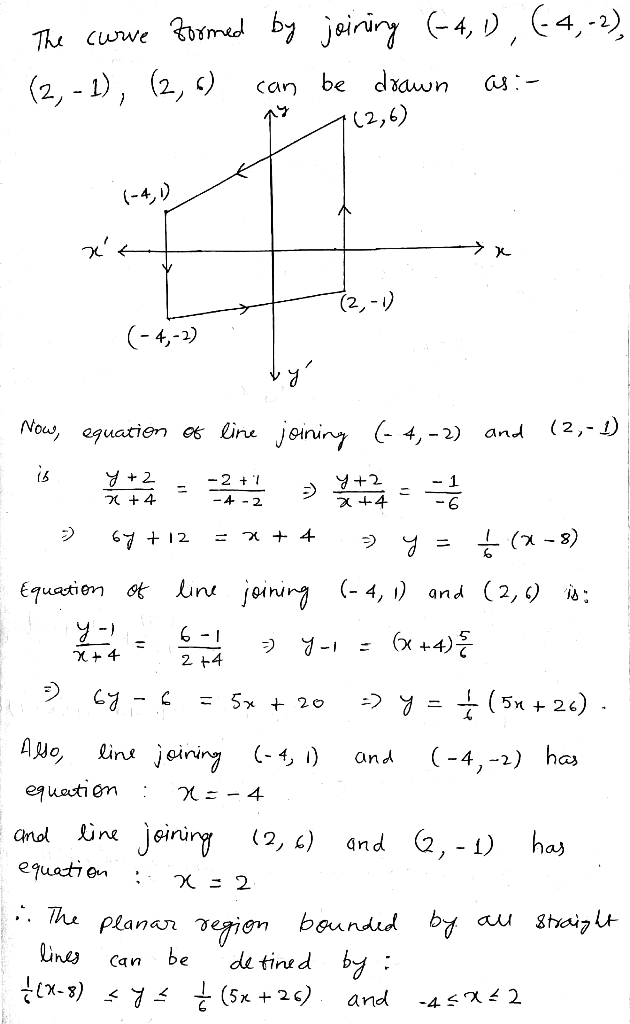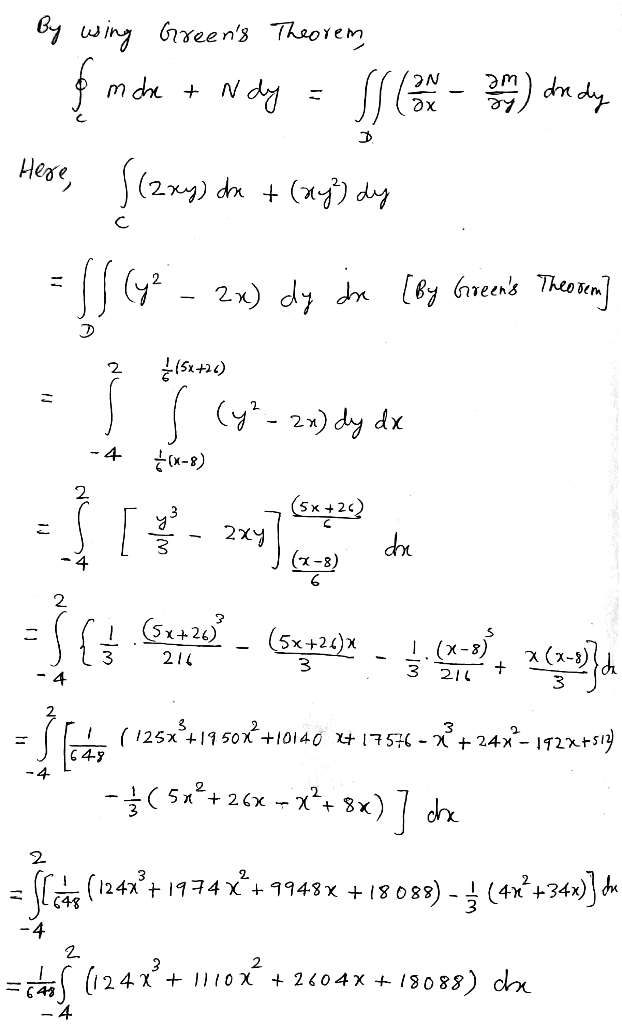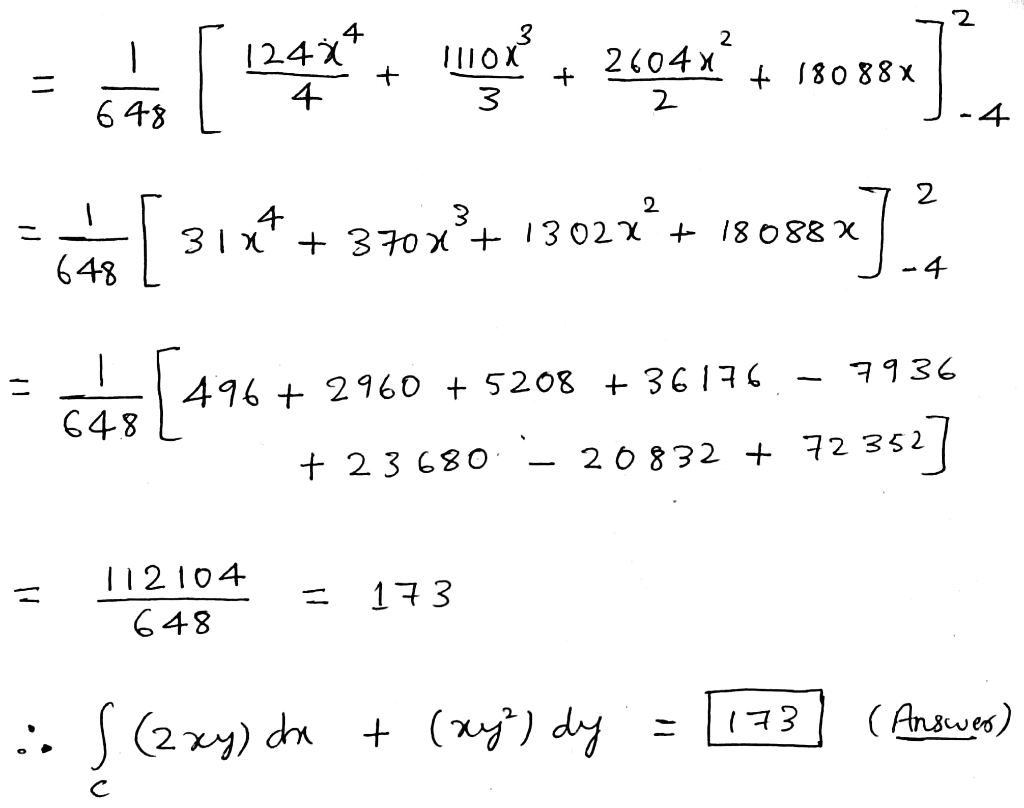# Let C be the closed, piecewise smooth curve formed by traveling in straight lines between the points (−4, 1), (−4, −2),...

Let C be the closed, piecewise smooth curve formed by traveling in straight lines between the points (−4, 1), (−4, −2), (2, −1), (2, 6), and back to (−4, 1), in that order. Use Green's theorem to evaluate the following integral. C (2xy) dx + (xy^2) dy##### Add Answer of: Let C be the closed, piecewise smooth curve formed by traveling in straight lines between the points (−4, 1), (−4, −2),...
More Homework Help Questions Additional questions in this topic.